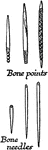### Reindeer age articles, Bone points and needles

Bone points and bone needles. Crafted during the Reindeer age.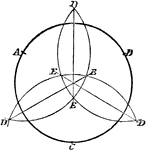### Construction of Circle Given 3 Points

Illustration used to construct a circle when given three points.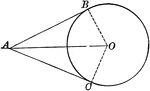### Circle With Two Tangents Drawn From an External Point

Illustration of a circle which illustrates that the tangents to a circle drawn from an external point…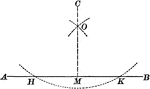### Construction of Perpendicular Upon a Given Line From an External Point

Illustration to let fall a perpendicular upon a given line from a given external point.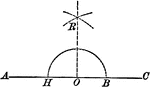### Construction of Perpendicular From a Given Point on a Straight Line

Illustration of the construction of a perpendicular to a line when given a point O on the straight line.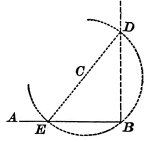### Construction of Perpendicular From a Given Point on a Straight Line

Illustration of the construction of a perpendicular to a line when given a point B on the straight line.### Celestial Crown

"CELESTIAL CROWN. Distinguished from any other crown by the stars on the points or rays that proceed…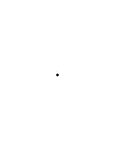### Flashcard of a Point

A flashcard featuring an illustration of a Point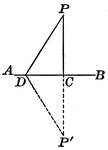### Perpendicular Line Drawn To a Given Line From an External Point

Illustration showing that the perpendicular is the shortest line that can be drawn to a straight line…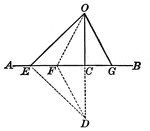### Lines Drawn From the Same Point in a Perpendicular to a Given line, Cutting Off Segments

Illustration showing two straight lines drawn from the same point in a perpendicular to a given line,…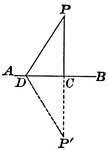### Perpendicular Line Drawn to a Given Line from an External Point

Illustration showing only one perpendicular can be drawn to a given line from a given external point.### Parallel Lines Cut by Transversals

Illustration that shows if two parallel lines are cut by three or more transversals that pass through…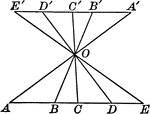### Parallel Lines Cut by Transversals

Illustration that shows if two parallel lines are cut by three or more transversals that pass through…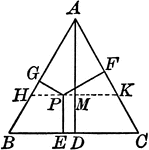### Perpendiculars Dropped Any Point in the Equilateral Triangle

Illustration to show the perpendiculars dropped from any point in the equilateral triangle to the three…